• 欢迎访问极客猴，分享 Python 入门，网络爬虫，数据分析，赚钱思维相关的技术与思维`QQ群`
• 本站点文章首发于微信公众号【极客猴】，欢迎关注，第一时间接受文章推送~
• 如果您觉得本站非常有看点，那么赶紧使用 Ctrl+D 收藏极客猴吧

# Python 绘图,我只用 Matplotlib(二)

5年前 (2017-11-06) 254次浏览

## 1.基础知识

### 1-1.库

``````import matplotlib.pyplot as plt   # 导入模块 matplotlib.pyplot，并简写成 plt
import numpy as np                # 导入模块 numpy，并简写成 np``````

numpy 是 Python 用于数学运算的库，它是在安装 matplotlib 时候顺带安装的。pyplot 是 matplotlib 一个子模块，主要为底层的面向对象的绘图库提供状态机界面。状态机隐式地自动创建数字和坐标轴以实现所需的绘图。 matplotlib 中的所有内容都按照层次结果进行组织。顶层就是由 pyplot 提供的 matplotlib “状态机环境”。基于这个状态机环境，我们就可以创建图形。

### 1-2.图形组成标签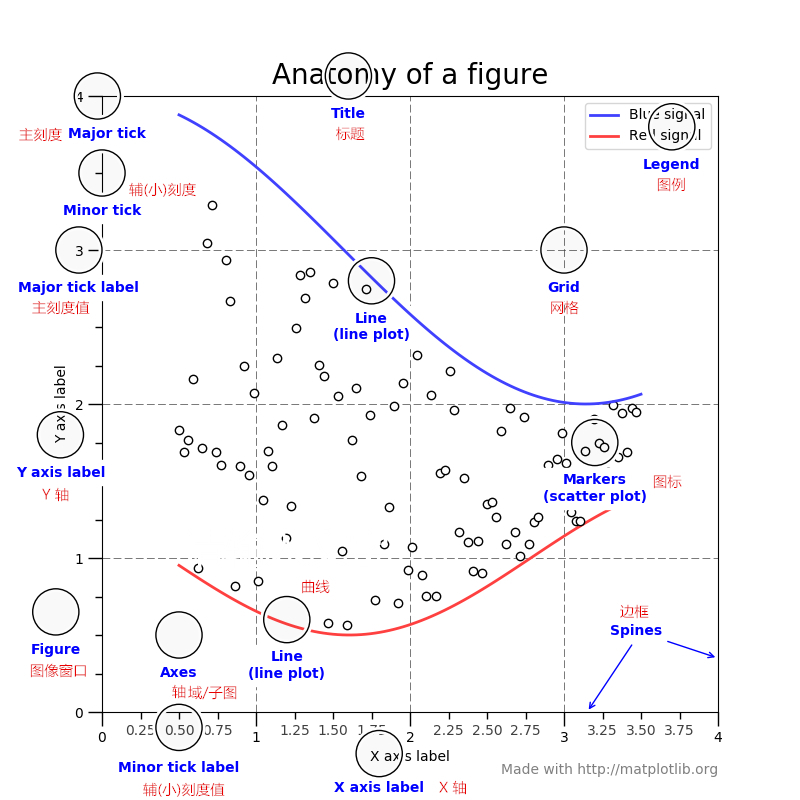• Figure

Figure 翻译成中文是图像窗口。Figure 是包裹 Axes、tiles、legends 等组件的最外层窗口。它其实是一个 Windows 应用窗口 。

Figure 中最主要的元素是 Axes（子图）。一个 Figure 中可以有多个子图，但至少要有一个能够显示内容的子图。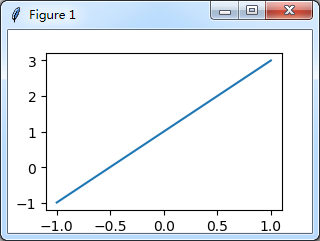• Axes

Axes 翻译成中文是轴域/子图。Axes 是带有数据的图像区域。从上文可知，它是位于 Figure 里面。那它和 Figure 是什么关系？这里可能文字难以表述清楚，我以图说文。用两图带你彻底弄清它们的关系。

``````fig = plt.figure()                      # 创建一个没有 axes 的 figure
fig.suptitle('No axes on this figure')  # 添加标题以便我们辨别

fig, ax_lst = plt.subplots(2, 2)        # 创建一个以 axes 为单位的 2x2 网格的 figure
plt.show()``````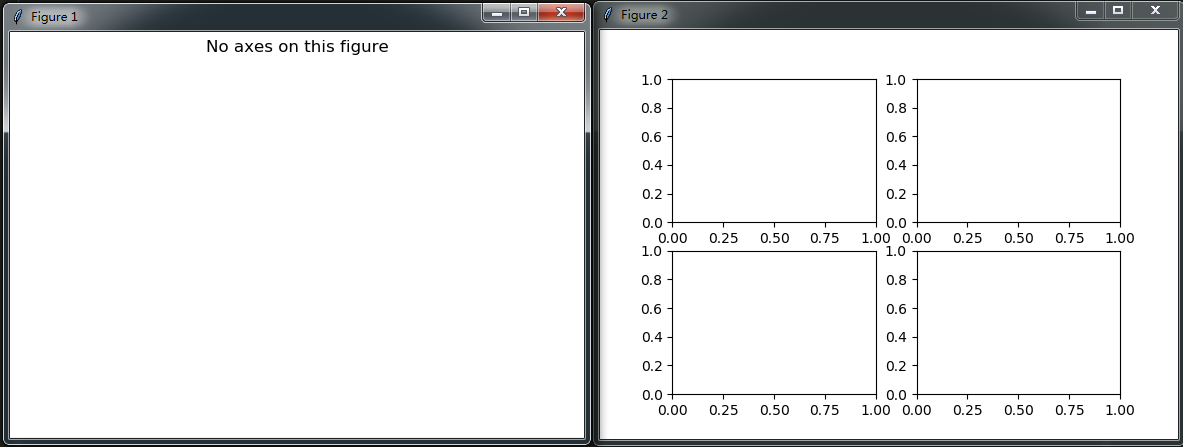• Axis

Axis 在中文的意思是轴。官网文档对 Axis 定义解释不清楚，让我们看得云里雾里的。如果你有留意前文的组成说明图，可以看到 X Axis 和 Y Axis 的字样。按照平常人的见识， 观察该图就能明白 Axis 是轴的意思。此外，Axis 和 Axes 以及 Figure 这三者关系，你看完下图，会恍然大悟。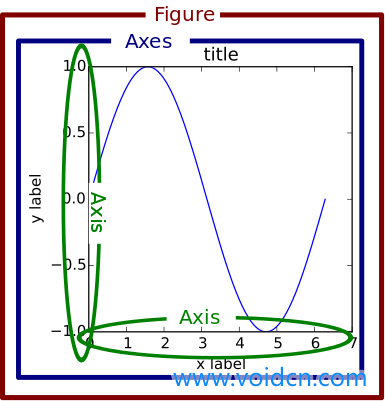## 2.绘制第一张图

### 2-1.初步绘制曲线

``````import matplotlib.pyplot as plt
import numpy as np

x = np.linspace(-2, 6, 50)
y1 = x + 3      # 曲线 y1
y2 = 3 - x      # 曲线 y2
plt.figure()    # 定义一个图像窗口
plt.plot(x, y1) # 绘制曲线 y1
plt.plot(x, y2) # 绘制曲线 y2
plt.show()``````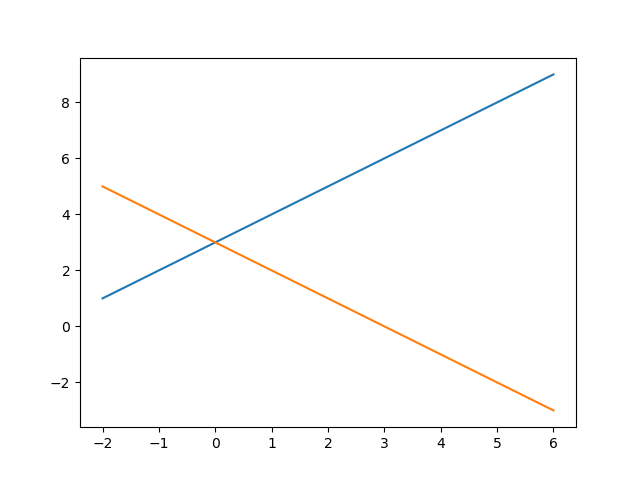### 2-2.简单修饰

``````import matplotlib.pyplot as plt
import numpy as np

# 创建一个点数为 8 x 6 的窗口, 并设置分辨率为 80像素/每英寸
plt.figure(figsize=(8, 6), dpi=80)

# 再创建一个规格为 1 x 1 的子图
plt.subplot(1，1，1)

x = np.linspace(-2, 6, 50)
y1 = x + 3        # 曲线 y1
y2 = 3 - x        # 曲线 y2

# 绘制颜色为蓝色、宽度为 1 像素的连续曲线 y1
plt.plot(x, y1, color="blue", linewidth=1.0, linestyle="-")
# 绘制颜色为紫色、宽度为 2 像素的不连续曲线 y2
plt.plot(x, y2, color="#800080", linewidth=2.0, linestyle="--")

# 设置横轴的上下限
plt.xlim(-1, 6)
# 设置纵轴的上下限
plt.ylim(-2, 10)

plt.show()``````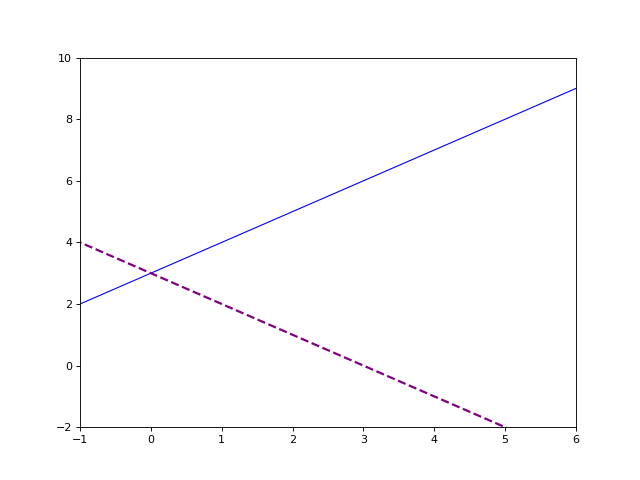### 2-3.设置纵横轴标签

``````...
# 设置横轴标签
plt.xlabel("X")
# 设置纵轴标签
plt.ylabel("Y")

plt.show()``````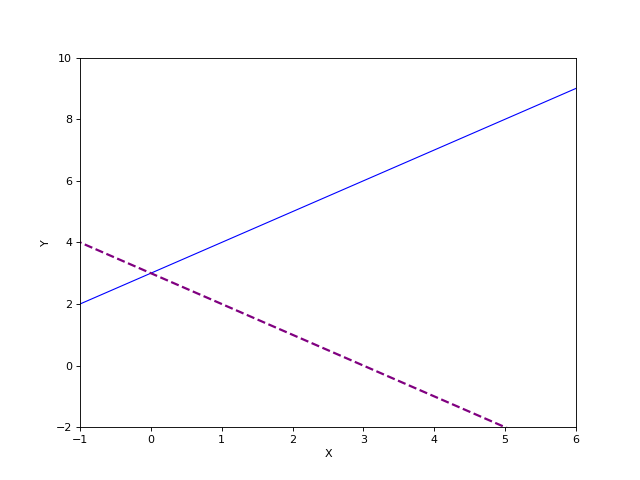### 2-4.设置精准刻度

matplotlib 画图设置的刻度是由曲线以及窗口的像素点等因素决定。这些刻度精确度无法满足需求，我们需要手动添加刻度。上图中，纵轴只显示 2 的倍数的刻度，横轴只显示 1 的倍数的刻度。我们为其添加精准刻度，纵轴变成单位间隔为 1 的刻度，横轴变成单位间隔为 0.5 的刻度。

``````...
# 设置横轴精准刻度
plt.xticks([-1, -0.5, 0, 0.5, 1, 1.5, 2, 2.5, 3, 3.5, 4, 4.5, 5, 5.5])
# 设置纵轴精准刻度
plt.yticks([-2, -1, 0, 1, 2, 3, 4, 5, 6, 7, 8, 9])

plt.show()``````

xticks() 和 yticks() 需要传入一个列表作为参数。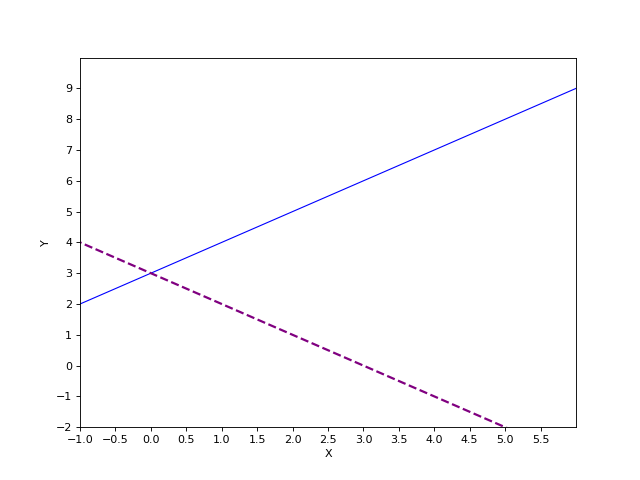``````...
# 设置横轴精准刻度
plt.xticks([-1, 0, 1, 2, 3, 4, 5, 6],
["-1m", "0m", "1m", "2m", "3m", "4m", "5m", "6m"])
# 设置纵轴精准刻度
plt.yticks([-2, 0, 2, 4, 6, 8, 10],
["-2m", "0m", "2m", "4m", "6m", "8m", "10m"])
plt.show()``````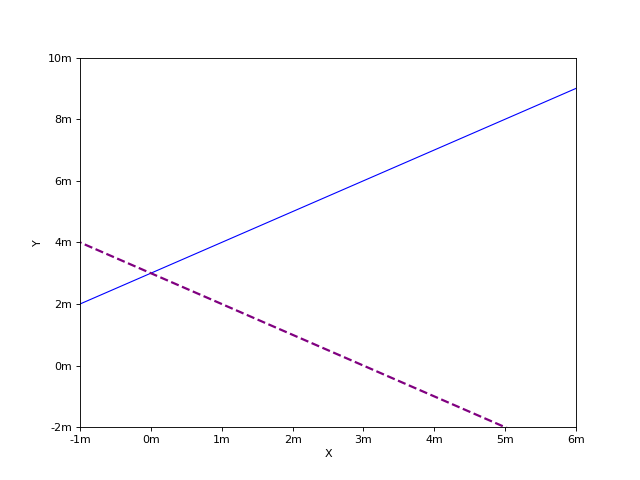### 2-5.添加图例

best 自动选择最佳位置，默认是左上
upper right 右上
upper left 左上
lower right 右下
lower left 左下
right 右边，默认是右上。如果因图形挡住右上，会自动往下选择空白地方绘制
center right 垂直居中且靠右
center left 垂直居中且靠左
lower center 垂直居中且靠底部
upper center 垂直居中且靠顶部
center 居中
``````...
# 绘制颜色为蓝色、宽度为 1 像素的连续曲线 y1
plt.plot(x, y1, color="blue", linewidth=1.0, linestyle="-", label="y1")
# 绘制颜色为紫色、宽度为 2 像素的不连续曲线 y2
plt.plot(x, y2, color="#800080", linewidth=2.0, linestyle="--", label="y2")
plt.legend(loc="upper left")
...``````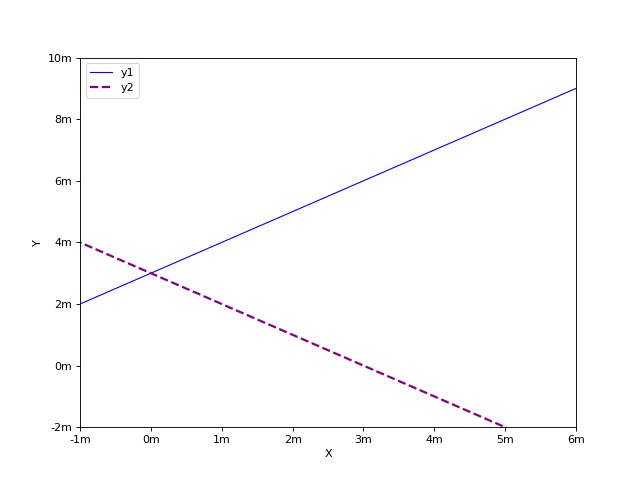### 2-6.注释特殊点位

`scatter()` 函数必须传入两个参数 x 和 y。值得注意得是，它们的数据类型是列表。x 代表要标注点的横轴位置，y 代表要标注点的横轴位置。x 和 y 列表中下标相同的数据是对应的。例如 x 为 [3, 4]，y 为 [6, 8]，这表示会绘制点（3，6），（4， 8）。因此，x 和 y 长度要一样。

`annotate`函数同样也有两个必传参数，一个是标注内容，另一个是 xy。标注内容是一个字符串。xy 表示要在哪个位置（点）显示标注内容。xy 位置地选定。一般是在`scatter()` 绘制点附近，但不建议重合，这样会影响美观。

``````...
# 绘制颜色为蓝色、宽度为 1 像素的连续曲线 y1
plt.plot(x, y1, color="blue", linewidth=1.0, linestyle="-", label="y1")
# 绘制散点(3, 6)
plt.scatter(, , s=30, color="blue")      # s 为点的 size
# 对(3, 6)做标注
plt.annotate("(3, 6)",
xy=(3.3, 5.5),       # 在(3.3, 5.5)上做标注
fontsize=16,         # 设置字体大小为 16
xycoords='data')  # xycoords='data' 是说基于数据的值来选位置

# 绘制颜色为紫色、宽度为 2 像素的不连续曲线 y2
plt.plot(x, y2, color="#800080", linewidth=2.0, linestyle="--", label="y2")
# 绘制散点(3, 0)
plt.scatter(, , s=50, color="#800080")
# 对(3, 0)做标注
plt.annotate("(3, 0)",
xy=(3.3, 0),            # 在(3.3, 0)上做标注
fontsize=16,          # 设置字体大小为 16
xycoords='data')    # xycoords='data' 是说基于数据的值来选位置``````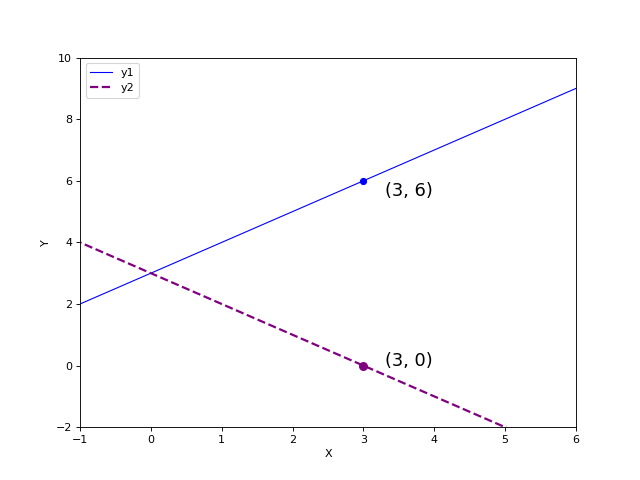``````···
# 绘制散点(3, 0)
plt.scatter(, , s=50, color="#800080")
# 对(3, 0)做标注
plt.annotate("(3, 0)", xy=(3.3, 0))
plt.text(4, -0.5, "this point very important",
fontdict={'size': 12, 'color': 'green'})  # fontdict设置文本字体``````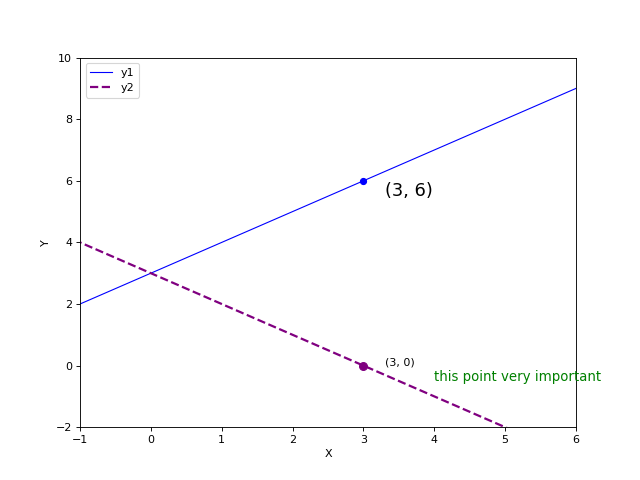[]